# Profit_splitter_contract

This project involves building smart contracts to automate some company finances to make everyone’s lives easier, increase transparency, and to make accounting and auditing practically automatic!

The profit splitter contracts will do the following:

• Pay Associate-level employees quickly and easily.
• Distribute profits to different tiers of employees.
• Distribute company shares for employees in a “deferred equity incentive plan” automatically.

This project was created in Remix IDE and uses the Ganache development chain and MetaMask (localhost:8545)

## Associate Profit Splitter Contract

This contract has two main functions:

• Balance — This function should is set to `public view returns(uint)`, and must return the contract’s current balance. Since we should always be sending Ether to the beneficiaries, this function should always return 0. If it does not, the deposit function is not handling the remainders properly and should be fixed. This will serve as a test function of sorts.

• Deposit — This function is set to `public payable check`, ensuring that only the owner can call the function and transfers an amount of Ether to three different employees.

It is important to note that `uint` only contains positive whole numbers, and Solidity does not fully support float/decimals, so we must deal with a potential remainder at the end of this function since amount will discard the remainder during division. We do this by transfering the `msg.value - amount * 3` back to `msg.sender`. This will re-multiply the amount by 3, then subtract it from the `msg.value` to account for any leftover wei, and send it back to Human Resources.

### Testing the Contract

The contract is compiled and then deployed by connecting to Injected Web3. During deployment, we assign employee addresses.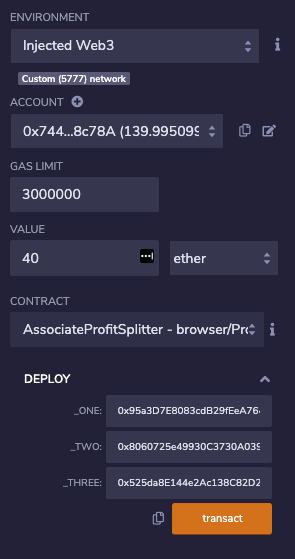Deposit of 40 Ether is executed through MetaMask: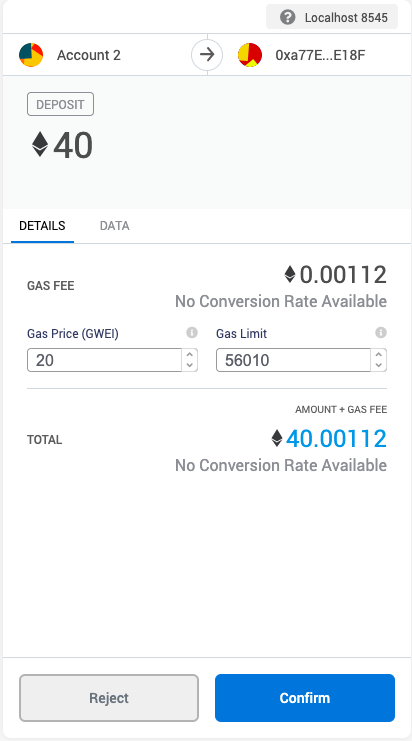Original Balances shown in Ganache: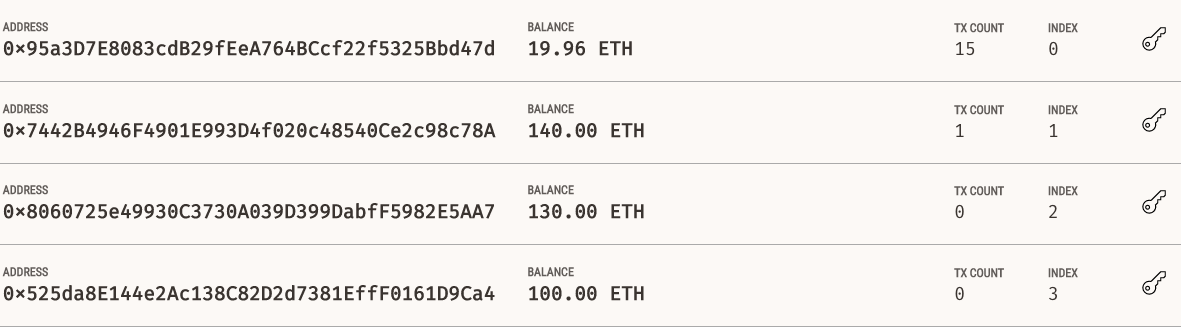Balances After Deposit shown in Ganache: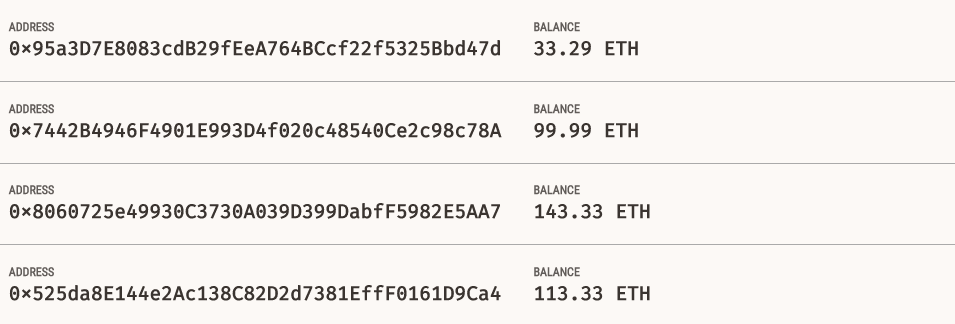Calling Balance Function:

Notice it returns a value of 0 which indicates are deposit function is handling the remainders successfully.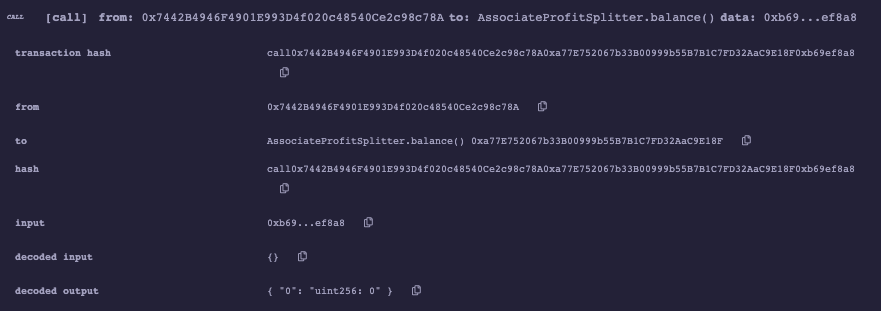## Tiered Profit Splitter Contract

In this contract, rather than splitting the profits between Associate-level employees, you will calculate rudimentary percentages for different tiers of employees (CEO, CTO, and Bob).

The deposit function has these differences:

• Calculation of the number of points/units is done by dividing `msg.value` by 100. This allows us to multiply the points with a number representing a percentage.
• The `uint amount` variable is used to store the amount to send each employee temporarily. For each employee, the amount is set equal to the number of points multiplied by the percentage
• For employee_one, distribute points * 60.
• For employee_two, distribute points * 25.
• For employee_three, distribute points * 15.
• After calculating the amount for the first employee, the amount is added to the total to keep a running total of how much of the `msg.value` has distributed.
• After transfering the amount, the remainder is sent to the employee with the highest percentage by subtracting `total` from `msg.value`.

## Deferred Equity Plan Contract

In this contract, we managed an employee’s “deferred equity incentive plan” in which 1000 shares will be distributed over 4 years to the employee. We didn’t work with Ether in this contract, but we store and set the amounts that represent the number of distributed shares the employee owns and enforce the vetting periods automatically.

For this contract, we performed the following:

• Human Resources was set in the constructor as the ‘msg.sender’, since HR will be deploying the contract.
• Total shares is set to 1000
• Annual distribution is set to 250. This equates to a 4 year vesting period for the total_shares, as 250 will be distributed per year.
• The `uint start_time = now;` line permanently stores the contract’s start date. We use this to calculate the vested shares later. Below this variable, the `unlock_time` is set equal to `now` plus `365 days`. We increment each distribution period.
• The `uint public distributed_shares` will track how many vested shares the employee has claimed and was distributed. By default, this is 0.

We use optional third-party analytics cookies to understand how you use GitHub.com so we can build better products. Learn more.

# Profit_splitter_contract

This project involves building smart contracts to automate some company finances to make everyone’s lives easier, increase transparency, and to make accounting and auditing practically automatic!

The profit splitter contracts will do the following:

• Pay Associate-level employees quickly and easily.
• Distribute profits to different tiers of employees.
• Distribute company shares for employees in a “deferred equity incentive plan” automatically.

This project was created in Remix IDE and uses the Ganache development chain and MetaMask (localhost:8545)

## Associate Profit Splitter Contract

This contract has two main functions:

• Balance — This function should is set to `public view returns(uint)`, and must return the contract’s current balance. Since we should always be sending Ether to the beneficiaries, this function should always return 0. If it does not, the deposit function is not handling the remainders properly and should be fixed. This will serve as a test function of sorts.

• Deposit — This function is set to `public payable check`, ensuring that only the owner can call the function and transfers an amount of Ether to three different employees.

It is important to note that `uint` only contains positive whole numbers, and Solidity does not fully support float/decimals, so we must deal with a potential remainder at the end of this function since amount will discard the remainder during division. We do this by transfering the `msg.value - amount * 3` back to `msg.sender`. This will re-multiply the amount by 3, then subtract it from the `msg.value` to account for any leftover wei, and send it back to Human Resources.

### Testing the Contract

The contract is compiled and then deployed by connecting to Injected Web3. During deployment, we assign employee addresses.Deposit of 40 Ether is executed through MetaMask:Original Balances shown in Ganache:Balances After Deposit shown in Ganache:Calling Balance Function:

Notice it returns a value of 0 which indicates are deposit function is handling the remainders successfully.## Tiered Profit Splitter Contract

In this contract, rather than splitting the profits between Associate-level employees, you will calculate rudimentary percentages for different tiers of employees (CEO, CTO, and Bob).

The deposit function has these differences:

• Calculation of the number of points/units is done by dividing `msg.value` by 100. This allows us to multiply the points with a number representing a percentage.
• The `uint amount` variable is used to store the amount to send each employee temporarily. For each employee, the amount is set equal to the number of points multiplied by the percentage
• For employee_one, distribute points * 60.
• For employee_two, distribute points * 25.
• For employee_three, distribute points * 15.
• After calculating the amount for the first employee, the amount is added to the total to keep a running total of how much of the `msg.value` has distributed.
• After transfering the amount, the remainder is sent to the employee with the highest percentage by subtracting `total` from `msg.value`.

## Deferred Equity Plan Contract

In this contract, we managed an employee’s “deferred equity incentive plan” in which 1000 shares will be distributed over 4 years to the employee. We didn’t work with Ether in this contract, but we store and set the amounts that represent the number of distributed shares the employee owns and enforce the vetting periods automatically.

For this contract, we performed the following:

• Human Resources was set in the constructor as the ‘msg.sender’, since HR will be deploying the contract.
• Total shares is set to 1000
• Annual distribution is set to 250. This equates to a 4 year vesting period for the total_shares, as 250 will be distributed per year.
• The `uint start_time = now;` line permanently stores the contract’s start date. We use this to calculate the vested shares later. Below this variable, the `unlock_time` is set equal to `now` plus `365 days`. We increment each distribution period.
• The `uint public distributed_shares` will track how many vested shares the employee has claimed and was distributed. By default, this is 0.

We use optional third-party analytics cookies to understand how you use GitHub.com so we can build better products. Learn more.

www.interchains.cc

https://www.interchains.cc/18321.html